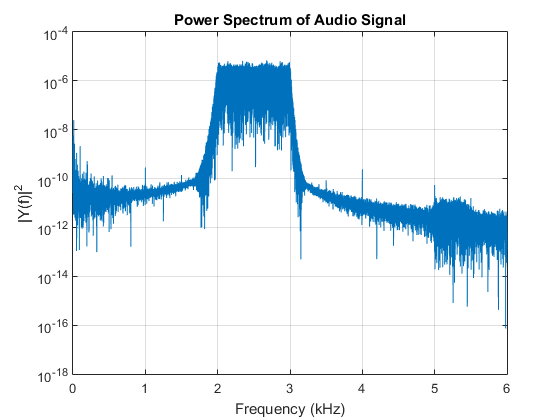## ARM CMIS IIR filters

There seems to be little information on the web about using Matlab and ARM CMSIS IIR functions. In princple it should be easy to design a filter in Matlab and run it on an an ARM chip. Well it is, its just the information seems to be spread over a number documents.

The design steps are as follows. Use Matlab to design an IIR filter and export the filter to the work space. (Or use any other method but convert it to SOS form). The format the CMSIS is expecting is slightly different to Matlab. Here is an example bandpass filter:

```            SOS =

1.0000   -0.9417    1.0000    1.0000    0.1845    0.8716
1.0000    1.7675    1.0000    1.0000    1.2434    0.9009
1.0000   -1.7449    1.0000    1.0000    0.5365    0.8054
1.0000    1.9538    1.0000    1.0000    0.9467    0.8240
1.0000   -0.6225    1.0000    1.0000    1.3914    0.9702
1.0000    1.6693    1.0000    1.0000    0.0035    0.9582
G =

0.6502
0.6502
0.8174
0.8174
0.0694
0.0694
1.0000
```

Each row of SOS is a stage and the parameters as `[b0,b1,b2,a0,a1,a2]`

Note `a0` is always one and is not needed. Looking at the Biquad Cascade IIR Filters Using Direct Form I Structure page we see the formula for each stage is `y[n] = b0 * x[n] + b1 * x[n-1] + b2 * x[n-2] + a1 * y[n-1] + a2 * y[n-2]`, which differs from Matlab's `y[n] = b0 * x[n] + b1 * x[n-1] + b2 * x[n-2] - a1 * y[n-1] - a2 * y[n-2]`. To correct for this we simply mutliple `a1`, and `a2` by -1. However, this also ignores the gain on each stage. To correct for this multiply `b1,b2,b3` by the stage gain value in `G`.

The new coefficent matrix that is in the correct format form CMSIS is now

```  Coeff =

0.6502   -0.6123    0.6502   -0.1845   -0.8716
0.6502    1.1491    0.6502   -1.2434   -0.9009
0.8174   -1.4262    0.8174   -0.5365   -0.8054
0.8174    1.5970    0.8174   -0.9467   -0.8240
0.0694   -0.0432    0.0694   -1.3914   -0.9702
0.0694    0.1159    0.0694   -0.0035   -0.9582
```

Note how `a0` has been removed. To automate this I have created a simple Matlab file which produces the header file.

An example snippet of C code is here

```#include "test.h"   //the file generated by MatlabSOS2CMSIS
float32_t pState[NUM_SECTIONS*4]={0};  //NUM_SECTIONS is define in test.h
arm_biquad_casd_df1_inst_f32 S;   //structure the defines the filter
```

In the your main function init the filter.

```arm_biquad_cascade_df1_init_f32(&S,NUM_SECTIONS,pCoeffs,pState);
```

To run the filter:

`arm_biquad_cascade_df1_f32(&S,pSrc,pDest,BUFFER_SIZE);`

See CMSIS doc for varible descriptionsThe figure above shows the response curve of the filter. This was generated by passing random white noise into the filter, converting it to audio and capturing via a PC sound card and FFTing in Matlab.